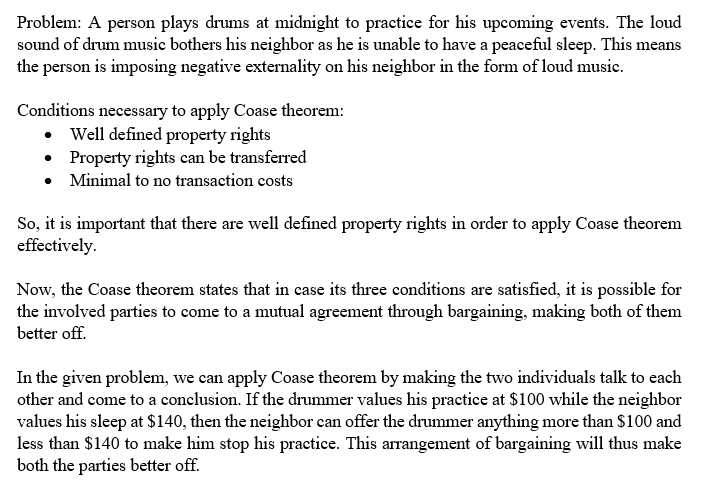In: Economics

# Describe an example of a problem that could be solved using the Coase theorem. Do this...

Describe an example of a problem that could be solved using the Coase theorem. Do this by defining a hypothetical situation where one individual is causing a negative externality on another individual. Describe whether it matters who holds property rights. Define the three necessary conditions for Coase theorem to work completely. Describe why a failure of these conditions will likely cause Coasian bargaining to fail.

## Solutions

##### Expert Solution## Related Solutions

##### Describe a negative externality that may (in theory) be solved by applying the Coase Theorem. a....
Describe a negative externality that may (in theory) be solved by applying the Coase Theorem. a. Explain what the Coase Theorem states and how it would be applied. b. Discuss 2-3 reasons why applying the Coase Theorem would be difficult in practice. Explain within the context of your example if possible or use a more general example.
##### What is an example of a problem that you feel could be solved using a regression...
What is an example of a problem that you feel could be solved using a regression model? Try to think of one in your chosen career field or one that you have interest in solving. In your post, specify what the problem is that you’d like to solve and how you would specify a model that would address the problem. What variables might impact on the outcome variable? Are they quantitative or categorical? You don’t have to actually collect data...
##### Explain using an example how the Coase Theorem states that by properly assigning property rights will...
Explain using an example how the Coase Theorem states that by properly assigning property rights will result in an efficient outcome (HINT: I highly recommend that you use a diagram to help explain your answer). What are the two assumptions needed for the Coase theorem to hold?
##### What is a business problem in business management that could be solved using a forecasting solution?...
What is a business problem in business management that could be solved using a forecasting solution? State what the issue is and how forecasting could aid in solving this problem, and the type of data that would be need to collect to solve this problem. Would there possibly be seasonal or trend factors in forecasts?
##### The Coase theorem implies that what
The Coase theorem implies that what
##### How could an assignment problem be solved using the transportation approach? What condition will make the...
How could an assignment problem be solved using the transportation approach? What condition will make the solution to this problem difficult? Please give a typed answer and focus on the second part of the question.
##### Which of the following uses the Coase Theorem to solve an externality problem? Select one: a....
Which of the following uses the Coase Theorem to solve an externality problem? Select one: a. A group of fishermen offer to pay a factory owner to reduce water pollution, even lower than the mandated level. b. The government taxes apple orchards to subsidize beekeepers. c. The government imposes fines for drunken driving. d. An apple orchard purchases the beehives on a neighboring farm.
##### What is the Coase theorem? Why is it not that practical?
What is the Coase theorem? Why is it not that practical?
##### What is         Coase Theorem?                 Why    does   private
What is         Coase Theorem?                 Why    does   private          solution         often fail      in            developing   countries?
##### Describe a problem that can be solved by using the shortest-route model. Give a detailed mathematical...
Describe a problem that can be solved by using the shortest-route model. Give a detailed mathematical example.